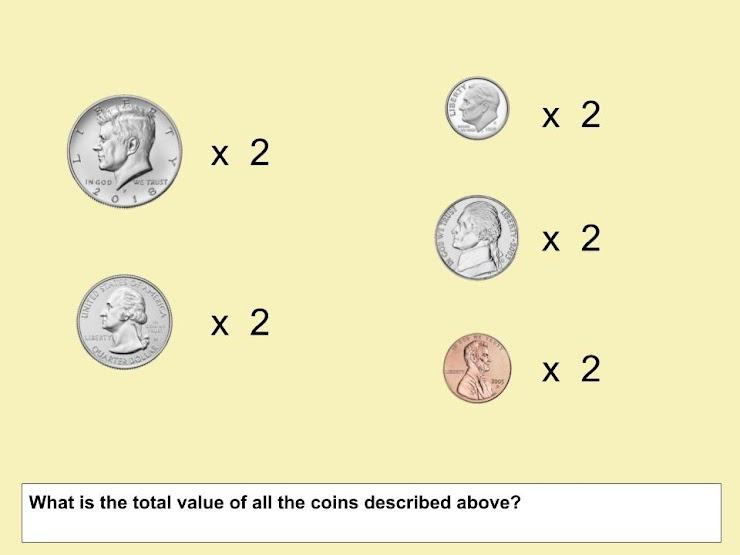Half-Dollars 1
(Set 1) Identify and know the value of coins, and show different combinations of coins that equal the same value. Students will see the cent sign ¢ and the dollar sign \$ in this activity. Half-Dollars, quarters, dimes, nickels & pennies only appear in this set, and the complexity of the coins being counted continues to increase.
From Mr. Anker Tests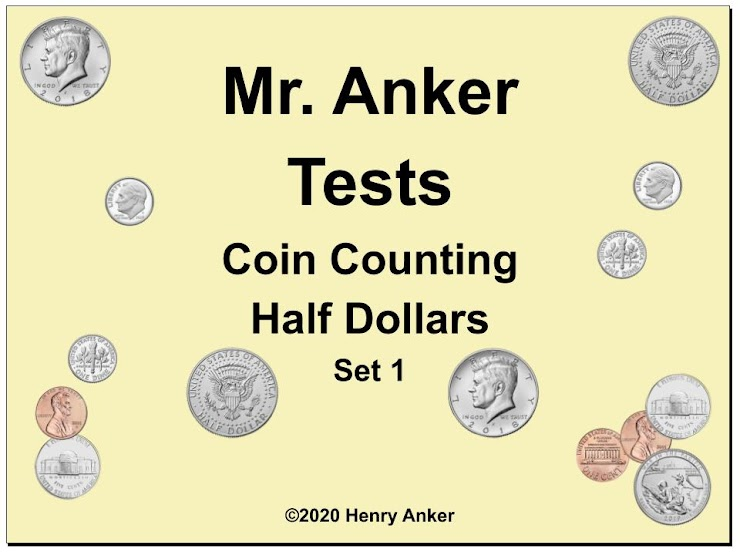Half-Dollars Help Slide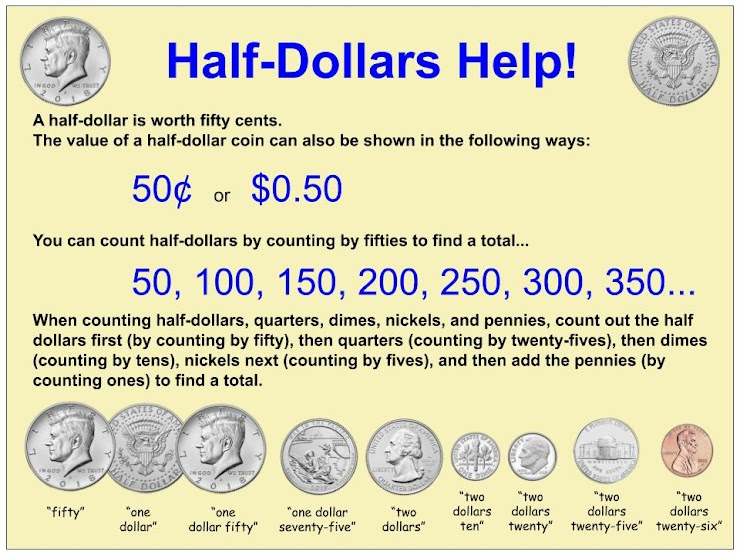1. What is the total value of the half-dollars shown below?
1 point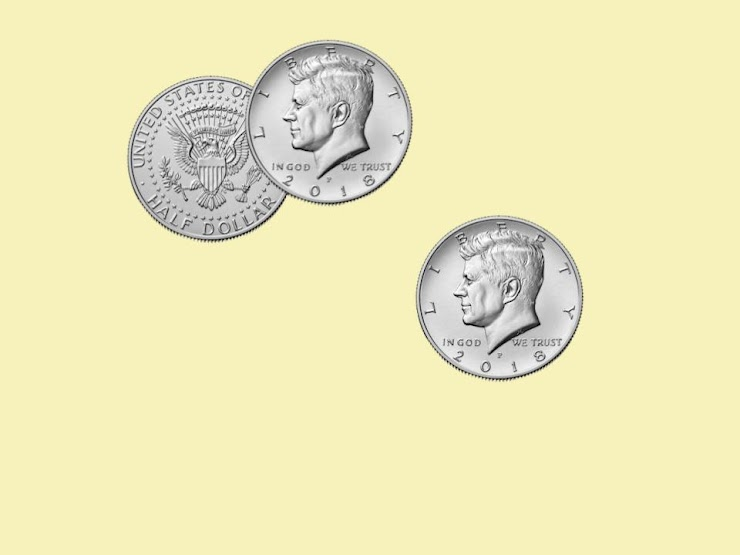2. What is the total value of the coins shown below?
1 point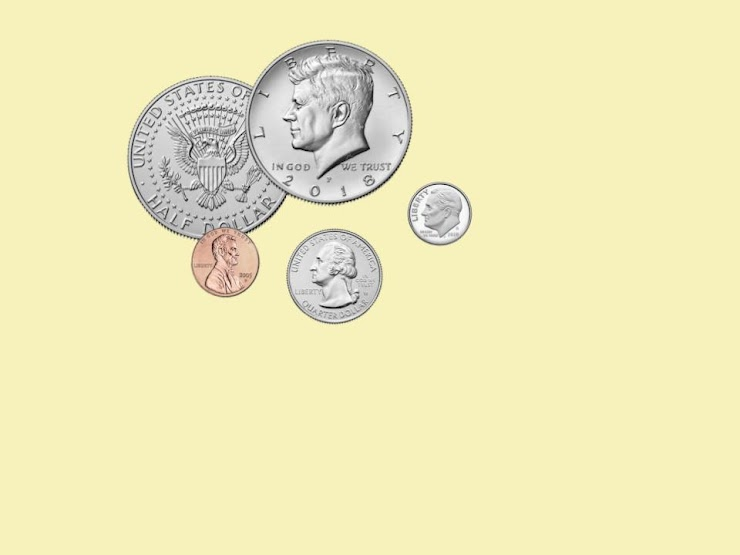3. What is the value of the coins shown below? (Be sure to use a dollar sign and a decimal point in your answer.)
1 point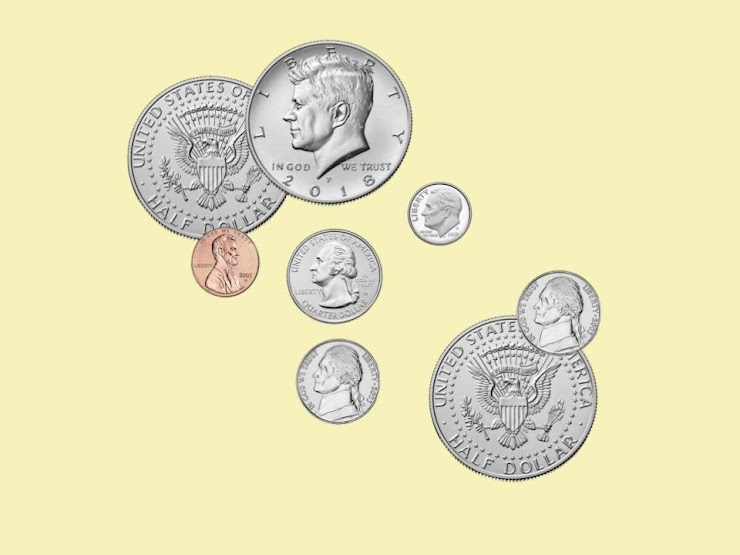4. What is the value of the coins shown below?
1 point5. What is the value of the coins shown below?
1 point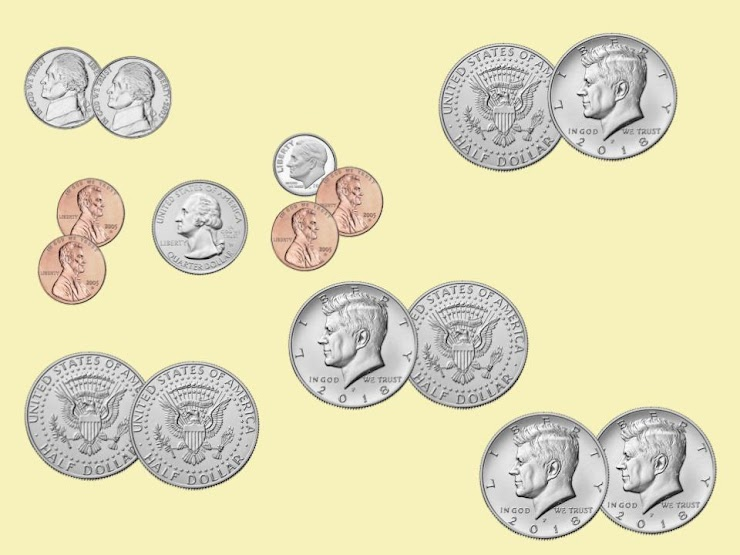6. What is the total value of the coins described here?
1 point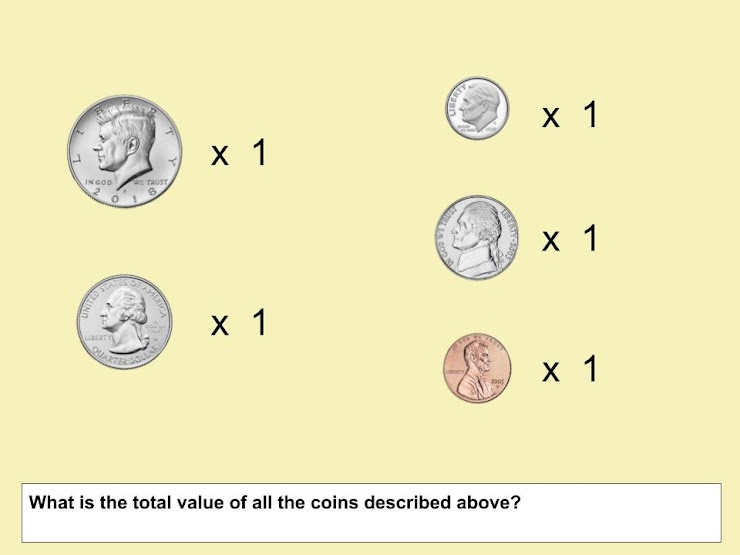7. What is the total value of the coins shown below?
1 point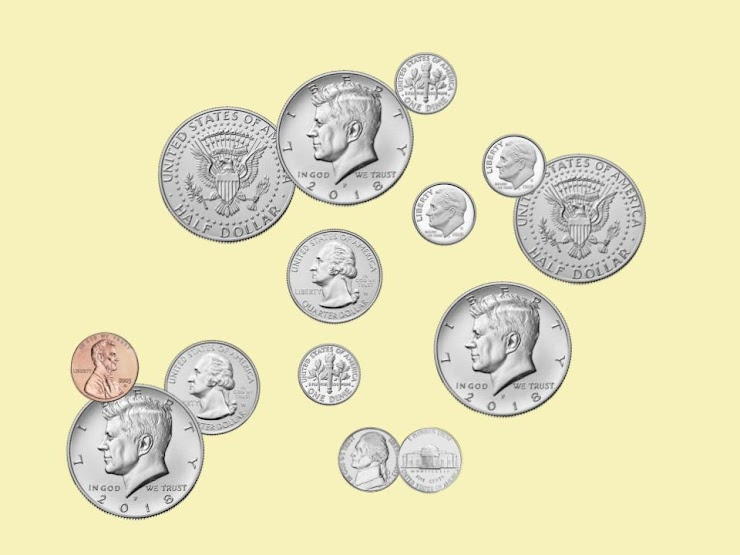8. Which two sets of coins have the same value?
1 point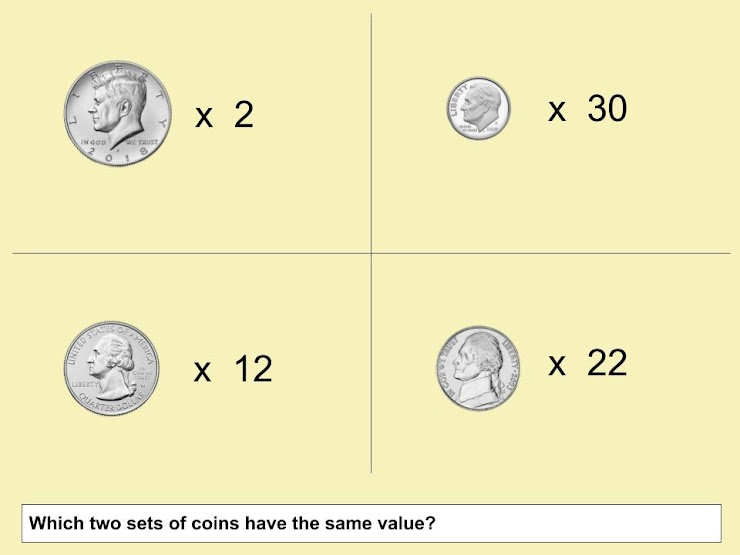9. Which two sets of coins have the same value?
1 point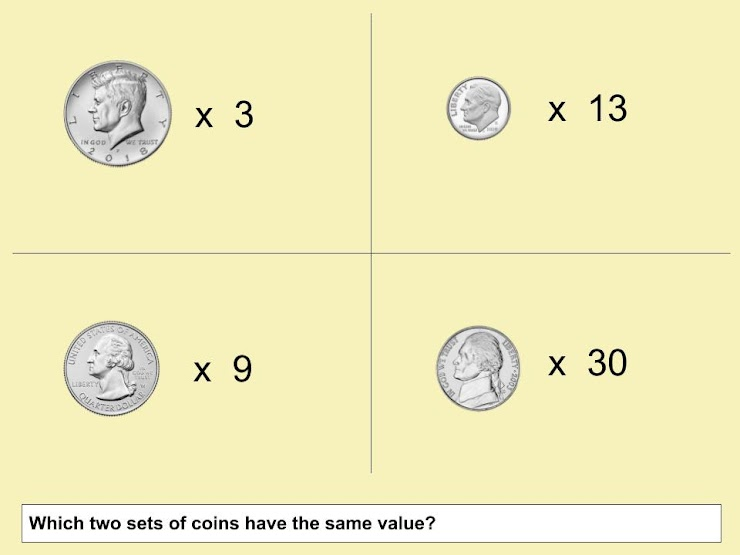10. What is the total value of the coins shown below?
1 point